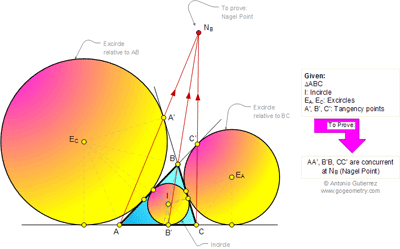## Wednesday, November 2, 2011

### Problem 684: Outer Nagel Point, Triangle, Excircles, Tangency points, Concurrent lines, Semi-perimeter, Ceva theorem

Geometry Problem
Level: Mathematics Education, High School, Honors Geometry, College.

Click the figure below to see the complete problem 684.1.AB':B'C = (s - a):(s - c)
CA':A'B = s:(s - a)
BC':C'A = (s - c): s
Product = 1
So AA', BB', CC' are concurrent (by Ceva)

2.Is there a solution using only projective geometry?